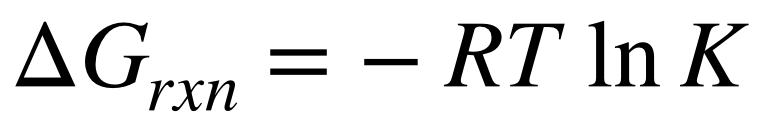# Problem: Consider the reaction and table of thermodynamic data at 298 K:What is the value of equilibrium constant for the reaction at 25  ̊C.a) 150b) 9.3 x 1015c) 8.4 x 104d) 1.1 x 10–16e) 1.4 x 108

###### FREE Expert Solution
87% (496 ratings)
###### FREE Expert Solution

Recall: The equilibrium constant (K) for any reaction is related to it's Gibb's free energy (ΔGrxn): [Eqn. 1]The Gibb's free energy of a reaction is also related to its enthalpy and entropy: [Eqn. 2]87% (496 ratings)###### Problem Details

Consider the reaction and table of thermodynamic data at 298 K:

What is the value of equilibrium constant for the reaction at 25  ̊C.

a) 150

b) 9.3 x 1015

c) 8.4 x 104

d) 1.1 x 10–16

e) 1.4 x 108What scientific concept do you need to know in order to solve this problem?

Our tutors have indicated that to solve this problem you will need to apply the Gibbs Free Energy concept. You can view video lessons to learn Gibbs Free Energy. Or if you need more Gibbs Free Energy practice, you can also practice Gibbs Free Energy practice problems.

What is the difficulty of this problem?

Our tutors rated the difficulty ofConsider the reaction and table of thermodynamic data at 298...as high difficulty.

How long does this problem take to solve?

Our expert Chemistry tutor, Dasha took 12 minutes and 56 seconds to solve this problem. You can follow their steps in the video explanation above.

What professor is this problem relevant for?

Based on our data, we think this problem is relevant for Professor Maxwell's class at UCF.